Over a million developers have joined DZone.{{announcement.body}}
{{announcement.title}}

DZone's Guide to

# Classification From Scratch Part 1 of 8: Logistic Regression

Let us start today our series on classification from scratch... The logistic regression is based on the assumption that given covariates , has a Bernoulli di...

· Big Data Zone ·
Free Resource

Comment (0)

Save
{{ articles.views | formatCount}} Views

Cloudera Data Flow, the answer to all your real-time streaming data problems. Manage your data from edge to enterprise with a no-code approach to developing sophisticated streaming applications easily. Learn more today.

Let us start today our series on classification from scratch ...

The logistic regression is based on the assumption that given covariates x, Y has a Bernoulli distribution,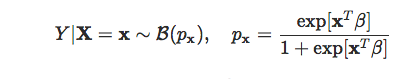The goal is to estimate the parameter of ß.

Recall that the heuristics for the use of that function for the probability is that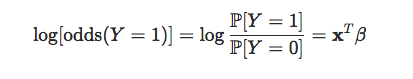## Maximum of the (log)-likelihood Function

The log-likelihood is here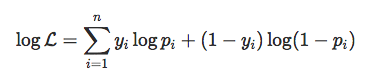where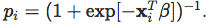Numerical techniques are based on (numerical) gradient descent to compute the maximum of the likelihood function. The (negative) log-likelihood is the following function:

``````y = myocarde\$PRONO
X = cbind(1,as.matrix(myocarde[,1:7]))
negLogLik = function(beta){
-sum(-y*log(1 + exp(-(X%*%beta))) - (1-y)*log(1 + exp(X%*%beta)))
}``````

We use the minus sign since standard optimization routines compute minima, not maxima. Now, to find the minimum of that function, we need a starting point to initiate the algorithm:

``beta_init = lm(PRONO~.,data=myocarde)\$coefficients``

Why not start with the parameter of the OLS. Somehow, we might think that at least sign should be ok, for instance. Anyway, we need a starting point, and let us use that one.

``logistic_opt = optim(par = beta_init, negLogLik, hessian=TRUE, method = "BFGS", control=list(abstol=1e-9))``

Here, we obtain:

``````logistic_opt\$par
(Intercept)        FRCAR        INCAR        INSYS
1.656926397  0.045234029 -2.119441743  0.204023835
PRDIA        PAPUL        PVENT        REPUL
-0.102420095  0.165823647 -0.081047525 -0.005992238``````

Let us verify here that this output is valid. For instance, what if we change the value of the starting point (randomly).

``````simu = function(i){
logistic_opt_i = optim(par = rnorm(8,0,3)*beta_init,
negLogLik, hessian=TRUE, method = "BFGS",
control=list(abstol=1e-9))
logistic_opt_i\$par[2:3]
}
v_beta = t(Vectorize(simu)(1:1000))
plot(v_beta)
par(mfrow=c(1,2))
hist(v_beta[,1],xlab=names(myocarde))
hist(v_beta[,2],xlab=names(myocarde))``````Ooops. There is a problem here. Clearly, we cannot rely on numerical optimization here. We can think about using another optimization routine:

``````library(optimx)
logit = function(mX, vBeta) {
exp(mX %*% vBeta)/(1+ exp(mX %*% vBeta))
}
logLikelihoodLogitStable = function(vBeta, mX, vY) {
-sum(vY*(mX %*% vBeta - log(1+exp(mX %*% vBeta))) +
(1-vY)*(-log(1 + exp(mX %*% vBeta))))
}
likelihoodScore = function(vBeta, mX, vY) {
return(t(mX) %*% (logit(mX, vBeta) - vY) )
}
optimLogitLBFGS = optimx(beta_init, logLikelihoodLogitStable,
method = 'L-BFGS-B', gr = likelihoodScore,
mX = X, vY = y, hessian=TRUE)``````

The optimum is:

``````attr(optimLogitLBFGS, "details")[]
[,1]
0.066680272
FRCAR  0.003080542
INCAR  0.079031364
INSYS -0.001586194
PRDIA  0.040500697
PAPUL -0.041870705
PVENT -0.014162756
REPUL  0.195632244``````

Let's be honest here, I do not feel comfortable with those techniques. So, what happened here?

Here, the technique we use is based on the following idea,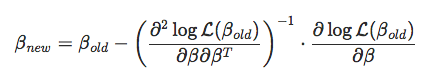The problem is that my computer does not know the first and second derivatives. So it will compute them using approximation techniques.

Actually, it is possible to use functions dedicated to such computations:

``````library(numDeriv)
library(MASS)
logit = function(x){1/(1+exp(-x))}
logLik = function(beta, X, y){
-sum(y*log(logit(X%*%beta)) +
(1-y)*log(1-logit(X%*%beta)))
}
optim_second = function(beta, num_iter){
LL = vector()
for(i in 1:num_iter){
grad = (t(X)%*%(logit(X%*%beta) - y))
H = hessian(logLik, beta, method = "complex", X = X, y = y)
beta = beta - ginv(H)%*%grad
LL[i] = logLik(beta, X, y)
}
result = list(beta, H)
return(result)
}``````

With our OLS starting point, we obtain:

``````opt0 = optim_second(beta_init,500)
opt0[]
[,1]
[1,]  0.951074420
[2,]  0.018860280
[3,]  0.275428978
[4,]  0.144803636
[5,] -0.058535606
[6,]  0.001182178
[7,] -0.108651776
[8,] -0.002940315``````

But if we try with another starting point:

``````opt1 = optim_second(beta_init*runif(8),500)
opt1[]
[,1]
[1,]  0.052894794
[2,]  0.024718435
[3,]  0.167953661
[4,]  0.171662947
[5,] -0.057458066
[6,] -0.011361034
[7,] -0.107532114
[8,] -0.002679064``````

Clearly, some coefficients are rather close. But others aren't. From my point of view, that is a major problem (keep in mind that we do not deal here with massive data! There are only 7 explanatory variables and only 71 observations).

Why not try to be clever, and use the analytical values of those derivatives? Even if some people claim the opposite, sometimes, it can actually be useful to do the math, instead of considering only numerical values.

## Newton (or Fisher) Algorithm

If you open any Econometrics textbooks (one can also try to derive it), you will get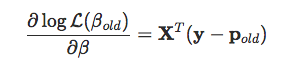while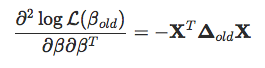``````Y=myocarde\$PRONO
X=cbind(1,as.matrix(myocarde[,1:7]))
colnames(X)=c("Inter",names(myocarde[,1:7]))
beta=as.matrix(lm(Y~0+X)\$coefficients,ncol=1)
for(s in 1:9){
pi=exp(X%*%beta[,s])/(1+exp(X%*%beta[,s]))
omega=matrix(0,nrow(X),nrow(X));diag(omega)=(pi*(1-pi))
Hessian=-t(X)%*%omega%*%X

Observe that here, I use only ten iterations of the algorithm!

`````` beta[,8:10]
[,1]          [,2]          [,3]
XInter -10.187641685 -10.187641696 -10.187641696
XFRCAR   0.138178119   0.138178119   0.138178119
XINCAR  -5.862429035  -5.862429037  -5.862429037
XINSYS   0.717084018   0.717084018   0.717084018
XPRDIA  -0.073668171  -0.073668171  -0.073668171
XPAPUL   0.016756506   0.016756506   0.016756506
XPVENT  -0.106776012  -0.106776012  -0.106776012
XREPUL  -0.003154187  -0.003154187  -0.003154187``````

The thing is that it seems to converge extremely fast. And it is rather robust! Look at what we get if we change our starting point:

``````beta=as.matrix(lm(Y~0+X)\$coefficients,ncol=1)*runif(8)
for(s in 1:9){
pi=exp(X%*%beta[,s])/(1+exp(X%*%beta[,s]))
omega=matrix(0,nrow(X),nrow(X));diag(omega)=(pi*(1-pi))
Hessian=-t(X)%*%omega%*%X
beta[,8:10]
[,1]          [,2]          [,3]
XInter -10.187641586 -10.187641696 -10.187641696
XFRCAR   0.138178118   0.138178119   0.138178119
XINCAR  -5.862429017  -5.862429037  -5.862429037
XINSYS   0.717084013   0.717084018   0.717084018
XPRDIA  -0.073668172  -0.073668171  -0.073668171
XPAPUL   0.016756508   0.016756506   0.016756506
XPVENT  -0.106776012  -0.106776012  -0.106776012
XREPUL  -0.003154187  -0.003154187  -0.003154187``````

Nice, isn't it? Looks like we got our winner, don't we? And one can use the inverse of the Hessian matrix to get standard deviations.

## Weighted Least-Squares

Let us go one step further. We've seen that we want to compute something like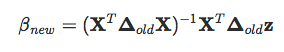(if we do substitute matrices in the analytical expressions) where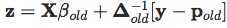But, actually, that's simply a standard least-square problem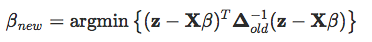The only problem here is that weights ∆old are functions of unknown ßold. But actually, if we keep iterating, we should be able to solve it: given the ß we got the weights, and with the weights, we can use weighted OLS to get an updated ß. That's the idea of iteratively reweighted least squares.

The algorithm will be:

``````df = myocarde
beta_init = lm(PRONO~.,data=df)\$coefficients
X = cbind(1,as.matrix(myocarde[,1:7]))
beta = beta_init
for(s in 1:1000){
p = exp(X %*% beta) / (1+exp(X %*% beta))
omega = diag(nrow(df))
diag(omega) = (p*(1-p))
df\$Z = X %*% beta + solve(omega) %*% (df\$PRONO - p)
beta = lm(Z~.,data=df[,-8], weights=diag(omega))\$coefficients
}``````

And the output is here:

``````beta
(Intercept)         FRCAR         INCAR         INSYS         PRDIA
-10.187641696   0.138178119  -5.862429037   0.717084018  -0.073668171
PAPUL         PVENT         REPUL
0.016756506  -0.106776012  -0.003154187``````

This is almost what we obtained before. Nice, isn't it? Actually, here, we also have standard deviations of estimators:

``````summary( lm(Z~.,data=df[,-8], weights=diag(omega)))

Coefficients:
Estimate Std. Error t value Pr(&gt;|t|)
(Intercept) -10.187642  10.668138  -0.955    0.343
FRCAR         0.138178   0.102340   1.350    0.182
INCAR        -5.862429   6.052560  -0.969    0.336
INSYS         0.717084   0.503527   1.424    0.159
PRDIA        -0.073668   0.261549  -0.282    0.779
PAPUL         0.016757   0.306666   0.055    0.957
PVENT        -0.106776   0.099145  -1.077    0.286
REPUL        -0.003154   0.004386  -0.719    0.475``````

## The Standard glm Function

Of course, it is possible to use an R built-in function to get our estimate:

``````summary(glm(PRONO~.,data=myocarde,family=binomial(link = "logit")))

Coefficients:
Estimate Std. Error z value Pr(&gt;|z|)
(Intercept) -10.187642  11.895227  -0.856    0.392
FRCAR         0.138178   0.114112   1.211    0.226
INCAR        -5.862429   6.748785  -0.869    0.385
INSYS         0.717084   0.561445   1.277    0.202
PRDIA        -0.073668   0.291636  -0.253    0.801
PAPUL         0.016757   0.341942   0.049    0.961
PVENT        -0.106776   0.110550  -0.966    0.334
REPUL        -0.003154   0.004891  -0.645    0.519``````

## Application and Visualization

Let us visualize the prediction obtained from the logistic regression, on our second dataset:

``````x = c(.4,.55,.65,.9,.1,.35,.5,.15,.2,.85)
y = c(.85,.95,.8,.87,.5,.55,.5,.2,.1,.3)
z = c(1,1,1,1,1,0,0,1,0,0)
df = data.frame(x1=x,x2=y,y=as.factor(z))
reg = glm(y~x1+x2,data=df,family=binomial(link = "logit"))
u = seq(0,1,length=101)
p = function(x,y) predict.glm(reg,newdata=data.frame(x1=x,x2=y),type="response")
v = outer(u,u,p)
image(u,u,v,xlab="Variable 1",ylab="Variable 2",col=clr10,breaks=(0:10)/10)
points(x,y,pch=19,cex=1.5,col="white")
points(x,y,pch=c(1,19)[1+z],cex=1.5)Here level curves - or iso-probabilities - are linear, so the space is divided in two (0 and 1, survival and death, white and black) by a straight line (or a hyperplane in higher dimensions). Furthermore, since we have a linear model, if we change the cutoff (the threshold used to create the two classes), we obtain another straight line (or hyperplane) parallel to the first one.

Next time, we will introduce splines to smooth those continuous covariates.

Cloudera Enterprise Data Hub. One platform, many applications. Start today.

Topics:
big data ,r ,regression analysis ,predictive analytics

Comment (0)

Save
{{ articles.views | formatCount}} Views

Published at DZone with permission of

Opinions expressed by DZone contributors are their own.

# {{ parent.title || parent.header.title}}

{{ parent.tldr }}

{{ parent.urlSource.name }}